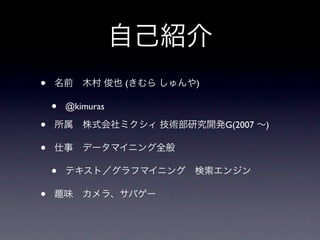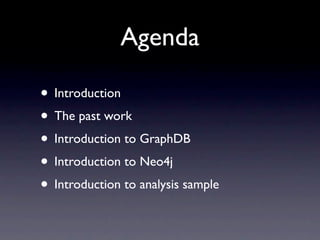Successfully reported this slideshow.

# ソーシャルグラフ分析

25

Share×
1 of 102
1 of 102

# ソーシャルグラフ分析

25

Share

About introduction to social graph analysis.

About introduction to social graph analysis.

## More Related Content

### Related Books

Free with a 14 day trial from Scribd

See all

### Related Audiobooks

Free with a 14 day trial from Scribd

See all

### ソーシャルグラフ分析

1. 1. • ( ) • @kimuras • G(2007 ) • • •
2. 2. Agenda • Introduction • The past work • Introduction to GraphDB • Introduction to Neo4j • Introduction to analysis sample
3. 3. Introduction
4. 4. Motivation for social graph analysis
5. 5. mixi 30000000 ID 22500000 # of member id 15000000 7500000 0 2007 2008 2009 2010 2011 year
6. 6. What is Social Graph?
7. 7. Feed Back
8. 8. Feed Back
9. 9. Feed Back
10. 10. Feed Back
11. 11. Feed Back
12. 12. Approach for SG analysis Feed Back
13. 13. The past work
14. 14. • •
15. 15. Relational Databases Dump & Denormalization from_id to_id id name age Key value 1 2 1 Kimura 18 From:1 2,3 1 3 2 kato 45 From:2 3 2 3 3 ito 21 Prof:1 Kimura,18 Prof:2 Kato,45
16. 16. Relational Databases Dump & reimplementation Denormalization from_id to_id id name age Key value 1 1 2 3 maintenance cost 1 2 Kimura kato 18 45 From:1 From:2 2,3 3 2 3 3 ito 21 Prof:1 Kimuras,18 Prof:2 Kato,45 scalability
17. 17. Introduction to GraphDB
18. 18. What is graph
19. 19. What is graph Vertex (node : )
20. 20. What is graph Vertex (node : ) Edge ( )
21. 21. What is graph Vertex (node : ) Undirected graph ( ) Edge ( )
22. 22. What is graph Vertex (node : ) Edge ( )
23. 23. What is graph Vertex (node : ) Edge ( )
24. 24. What is graph Vertex (node : ) Edge ( )
25. 25. What is graph Vertex (node : ) Directed graph ( ) Edge ( )
26. 26. What is GraphDB Vertex (node : ) Edge ( )
27. 27. What is GraphDB ID: 1 Vertex (node : ) NAME: kimura PROP: Male AGE: 18 Edge ( )
28. 28. What is GraphDB ID: 1 Vertex (node : ) NAME: kimura PROP: Male AGE: 18 Edge ( ) ID: 2 NAME: ITO PROP: Female AGE: 21
29. 29. What is GraphDB ID: 1 Vertex (node : ) NAME: kimura PROP: Male AGE: 18 Edge ( ) ID: 2 NAME: ITO PROP: Female AGE: 21
30. 30. What is GraphDB ID: 1 Vertex (node : ) NAME: kimura PROP: Male AGE: 18 Edge ( ) ID: 2 NAME: ITO PROP: Female AGE: 21
31. 31. What is GraphDB ID: 1 Vertex (node : ) NAME: kimura PROP: Male AGE: 18 Edge ( ) ID: 2 ID: 3 NAME: ITO LABEL: Like PROP: Female Since: 2011/08/06 AGE: 21 OutGoing: 2
32. 32. What is GraphDB ID: 1 Vertex (node : ) NAME: kimura PROP: Male AGE: 18 Edge ( ) ID: 2 ID: 3 NAME: ITO LABEL: Like PROP: Female Since: 2011/08/06 AGE: 21 OutGoing: 2
33. 33. What is GraphDB ID: 1 Vertex (node : ) NAME: kimura PROP: Male AGE: 18 Edge ( ) ID: 2 ID: 3 NAME: ITO LABEL: Like PROP: Female Since: 2011/08/06 AGE: 21 OutGoing: 2
34. 34. The implementations for GraphDB http://en.wikipedia.org/wiki/GraphDB
35. 35. Introduction to Neo4j
36. 36. GraphDB Neo4j • True ACID transactions • High availability • Scales to billions of nods and relationships • High speed querying through traversals Single instance(GPLv3) Multiple instance(AGPLv3) Embedded EmbeddedGraphDatabase HighlyAvailableGraphDatabase Standalone Neo4j Server Neo4j Server high availability mode http://neo4j.org/
37. 37. Other my favorite features for Neo4j • RESTful APIs • Query Language(Cypher) • Full indexing – lucene • Implemented graph algorithm – A*, Dijkstra – High speed traverse • Gremlin supported – Like a query language http://www.tinkerpop.com/post/4633229547/tinkerpop-graph-stack
38. 38. Introduction simple Neo4j usecase Single node Multi node Embedded Analyses system Analyses system Analyses system Analyses system Server
39. 39. Introduction simple Neo4j usecase Single node Multi node Embedded Analyses system Analyses system Analyses system Analyses system Server
40. 40. Introduction simple Neo4j usecase Single node Multi node Analyses system Embedded Analyses system Analyses system Analyses system Server
41. 41. Introduction simple Neo4j usecase Single node Multi node Analyses system Embedded Analyses system Analyses system Analyses system Server
42. 42. Introduction to simple embedded Neo4j • Insert Vertices & make Relationships • Single node & Embedded • Traversal sample
43. 43. Insert vertices, make relationship public final class InputVertex { public static void main(final String[] args) { GraphDatabaseService graphDb = new EmbeddedGraphDatabase("/tmp/neo4j"); Transaction tx = graphDb.beginTx(); try { Node firstNode = graphDb.createNode(); firstNode.setProperty("Name", "Kimura"); Node secondNode = graphDb.createNode(); secondNode.setProperty("Name", "Kato"); firstNode.createRelationshipTo(secondNode, DynamicRelationshipType.withName("LIKE")); tx.success(); } finally { tx.finish(); } graphDb.shutdown(); } }
44. 44. Insert vertices, make relationship public final class InputVertex { public static void main(final String[] args) { GraphDatabaseService graphDb = new EmbeddedGraphDatabase("/tmp/neo4j"); Transaction tx = graphDb.beginTx(); try { Node firstNode = graphDb.createNode(); firstNode.setProperty("Name", "Kimura"); Node secondNode = graphDb.createNode(); secondNode.setProperty("Name", "Kato"); firstNode.createRelationshipTo(secondNode, DynamicRelationshipType.withName("LIKE")); tx.success(); } finally { tx.finish(); } graphDb.shutdown(); } }
45. 45. Insert vertices, make relationship public final class InputVertex { public static void main(final String[] args) { ID: 1 GraphDatabaseService graphDb = new NAME: kimura EmbeddedGraphDatabase("/tmp/neo4j"); Transaction tx = graphDb.beginTx(); try { Node firstNode = graphDb.createNode(); firstNode.setProperty("Name", "Kimura"); Node secondNode = graphDb.createNode(); secondNode.setProperty("Name", "Kato"); firstNode.createRelationshipTo(secondNode, DynamicRelationshipType.withName("LIKE")); tx.success(); } finally { tx.finish(); } graphDb.shutdown(); } }
46. 46. Insert vertices, make relationship public final class InputVertex { public static void main(final String[] args) { ID: 1 GraphDatabaseService graphDb = new NAME: kimura EmbeddedGraphDatabase("/tmp/neo4j"); Transaction tx = graphDb.beginTx(); try { Node firstNode = graphDb.createNode(); firstNode.setProperty("Name", "Kimura"); Node secondNode = graphDb.createNode(); secondNode.setProperty("Name", "Kato"); firstNode.createRelationshipTo(secondNode, DynamicRelationshipType.withName("LIKE")); tx.success(); } finally { tx.finish(); } graphDb.shutdown(); } }
47. 47. Insert vertices, make relationship public final class InputVertex { public static void main(final String[] args) { ID: 1 GraphDatabaseService graphDb = new NAME: kimura EmbeddedGraphDatabase("/tmp/neo4j"); Transaction tx = graphDb.beginTx(); try { Node firstNode = graphDb.createNode(); firstNode.setProperty("Name", "Kimura"); Node secondNode = graphDb.createNode(); secondNode.setProperty("Name", "Kato"); firstNode.createRelationshipTo(secondNode, DynamicRelationshipType.withName("LIKE")); tx.success(); } finally { ID: 2 tx.finish(); NAME: Kato } graphDb.shutdown(); } }
48. 48. Insert vertices, make relationship public final class InputVertex { public static void main(final String[] args) { ID: 1 GraphDatabaseService graphDb = new NAME: kimura EmbeddedGraphDatabase("/tmp/neo4j"); Transaction tx = graphDb.beginTx(); try { Node firstNode = graphDb.createNode(); firstNode.setProperty("Name", "Kimura"); Node secondNode = graphDb.createNode(); secondNode.setProperty("Name", "Kato"); firstNode.createRelationshipTo(secondNode, DynamicRelationshipType.withName("LIKE")); tx.success(); } finally { ID: 2 tx.finish(); NAME: Kato } graphDb.shutdown(); } }
49. 49. Insert vertices, make relationship public final class InputVertex { public static void main(final String[] args) { ID: 1 GraphDatabaseService graphDb = new NAME: kimura EmbeddedGraphDatabase("/tmp/neo4j"); Transaction tx = graphDb.beginTx(); try { Node firstNode = graphDb.createNode(); ID: 3 firstNode.setProperty("Name", "Kimura"); Relation: Like Node secondNode = graphDb.createNode(); secondNode.setProperty("Name", "Kato"); firstNode.createRelationshipTo(secondNode, DynamicRelationshipType.withName("LIKE")); tx.success(); } finally { ID: 2 tx.finish(); NAME: Kato } graphDb.shutdown(); } }
50. 50. Batch Insert • Non thread safe, non transaction • But very fast! public final class Batch { public static void main(final String[] args) { BatchInserter inserter = new BatchInserterImpl("/tmp/neo4j", BatchInserterImpl.loadProperties("/tmp/neo4j.props")); Map<String, Object> prop = new HashMap<String, Object>(); prop.put("Name", "Kimura"); prop.put("Age", 21); long node1 = inserter.createNode(prop); prop.put("Name", "Kato"); prop.put("Age", 21); long node2 = inserter.createNode(prop); inserter.createRelationship(node1, node2, DynamicRelationshipType.withName("LIKE"), null); inserter.shutdown(); } }
51. 51. Traversal sample • public static void main(final String[] args) { GraphDatabaseService graphDB = new EmbeddedGraphDatabase(args); Node node = graphDB.getNodeById(1); Traverser friends = node.traverse( // Order.DEPTH_FIRST, BREADTH_FIRST // StopEvaluator.END_OF_GRAPH, DEPTH_ONE // ReturnableEvaluator.ALL_BUT_START_NODE, ALL, isReturnableNode() // DynamicRelationshipType.withName("LIKE"), // Direction.OUTGOING); INCOMING, BOTH for (Node nodeBuf : friends) { TraversalPosition currentPosition = friends.currentPosition(); } }
52. 52. Traversal sample Order.BREADTH_FIRST •
53. 53. Traversal sample Order.BREADTH_FIRST •
54. 54. Traversal sample Order.BREADTH_FIRST •
55. 55. Traversal sample Order.BREADTH_FIRST •
56. 56. Traversal sample Order.BREADTH_FIRST •
57. 57. Traversal sample Order.BREADTH_FIRST •
58. 58. Traversal sample Order.DEPTH_FIRST •
59. 59. Traversal sample Order.DEPTH_FIRST •
60. 60. Traversal sample Order.DEPTH_FIRST •
61. 61. Traversal sample Order.DEPTH_FIRST •
62. 62. Traversal sample Order.DEPTH_FIRST •
63. 63. Traversal sample Order.DEPTH_FIRST •
64. 64. Neoclipse sample http://wiki.neo4j.org/content/Neoclipse
65. 65. experiment • mixi Neo4j • • Machine: 24 core CPU, Memory 65GB • Neo4j: BatchInsert, community, embedded • Data • 1500 6 513m17sec (about 8.6h)
66. 66. Network Dataset • Stanford Large Network Dataset Collection • SNAP has a Wide variety of graph data! Social Networks Communication networks Citation networks Collaboration networks Web graphs Product co-purchasing networks Internet peer-to-peer networks Road networks Autonomous systems graphs Signed networks Wikipedia networks and metadata Memetracker and Twitter http://snap.stanford.edu/data/index.html
67. 67. Introduction to Analysis Sample
68. 68. Architecture Service Database Analysis Visualization (Social Graph)
69. 69. Introduction Analyses Sample • Centrality ( ) • Clustering coefﬁcient ( )
70. 70. Centrality ( ) • = Pagerank
71. 71. • • = Vertex ( )
72. 72. • • = Vertex ( ) 1 1 1
73. 73. • • = Vertex ( ) 2 1 1 2 1 2
74. 74. • • = Vertex ( ) 2 1 1 2 1 2
75. 75. • • = Vertex ( ) 2 1 1 4 2 1 2
76. 76. • • = Vertex ( ) 2 1 1 4 2 1 2
77. 77. mixi • 1000 • summary Min 1st Que. Median Mean 3rd Que. Max 1.00 3.00 10.00 25.69 30.00 903.00
78. 78. • • ≒
79. 79. • • ≒ =0/3=0
80. 80. • • ≒ =0/3=0 =1/3
81. 81. • • ≒ =0/3=0 =1/3 =2/3
82. 82. • • ≒ =0/3=0 =1/3 =2/3 =3/3=1
83. 83. • 1000 • summary Min 1st Que. Median Mean 3rd Que. Max 0.00 0.00 0.1157 0.2071 0.2667 1.000
84. 84. • 25 0.08
85. 85. • 14 0.17
86. 86. • 10 0.68
87. 87. • 4 1
88. 88. Visualization Sample
89. 89. • 2hop Social Graph • Edge • ( ) • Vertex • ( ) • Gephi http://gephi.org/
90. 90. • Social Graph • • GraphDB • Neo4j • R • Visualization
91. 91. Thanks!

### Editor's Notes

• \n
• \n
• \n
• \n
• \n
• \n
• \n
• \n
• \n
• \n
• \n
• \n
• \n
• \n
• \n
• \n
• \n
• \n
• \n
• \n
• \n
• \n
• \n
• \n
• \n
• \n
• \n
• \n
• \n
• \n
• \n
• \n
• \n
• \n
• \n
• \n
• \n
• \n
• \n
• &amp;#x30FB;TC&amp;#x3082;mysql&amp;#x3082;&amp;#x73FE;&amp;#x5F79;&amp;#x3060;&amp;#x3057;&amp;#x3001;&amp;#x5927;&amp;#x597D;&amp;#x304D;\n
• &amp;#x30FB;TC&amp;#x3082;mysql&amp;#x3082;&amp;#x73FE;&amp;#x5F79;&amp;#x3060;&amp;#x3057;&amp;#x3001;&amp;#x5927;&amp;#x597D;&amp;#x304D;\n
• \n
• \n
• \n
• \n
• \n
• \n
• \n
• \n
• \n
• \n
• \n
• \n
• \n
• \n
• \n
• \n
• \n
• \n
• \n
• \n
• \n
• \n
• \n
• \n
• \n
• \n
• \n
• \n
• \n
• \n
• \n
• \n
• \n
• \n
• \n
• \n
• \n
• \n
• \n
• \n
• \n
• \n
• \n
• \n
• \n
• \n
• \n
• \n
• \n
• \n
• \n
• \n
• \n
• \n
• \n
• \n
• \n
• \n
• \n
• \n
• \n
• \n
• \n
• \n
• \n
• \n
• \n
• \n
• \n
• \n
• \n
• \n
• \n
• \n
• \n
• \n
• \n
• \n
• \n
• \n
• \n
• \n
• \n
• \n
• \n
• \n
• \n
• \n
• \n
• \n
• \n